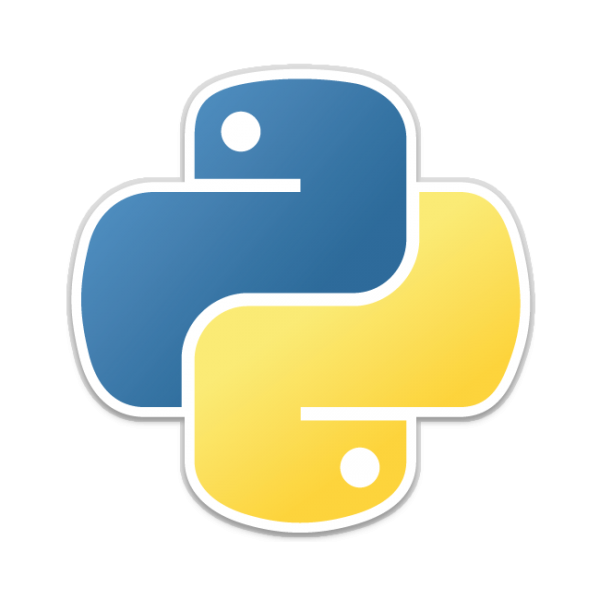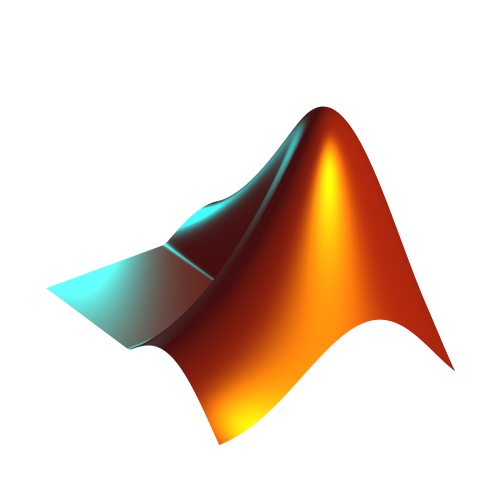# MATH 3090 Section A- Computational Mathematics

Fall 2018

Classes: Health, Nursing, and Environmental Studies Building (HNE) Room 037, Mondays Wednesdays, and Fridays 11:30am

email: msamani[at]fields.utoronto.ca

Office: South Ross 516

Office hours: Wednesdays 1pm - 3pm

Teaching Assistant: Nathan Gold
email: ngold5[at]my.yorku.ca

Pre-requisites: SC/MATH 2022 3.00; SC/MATH 2030 3.00; LE/CSE 1560 3.00, or LE/CSE 2031 3.00 and SC/MATH 2041 3.00, or LE/CSE 1540 3.00 and SC/MATH 2041 3.00.
PRIOR TO SUMMER 2013: Prerequisites: SC/MATH 2022 3.00; SC/MATH 2030 3.00; SC/CSE 1560 3.00, or SC/CSE 2031 3.00 and SC/MATH 2041 3.00, or SC/CSE 1540 3.00 and SC/MATH 2041 3.00.

Course outline: We cover various computational techniques including methods for solving systems of linear equations, ordinary and partial differential equations, Fourier analysis, and random number generation. We talk about fitting curves to data, and interpreting the results. We also develop strategies for modeling simple physical processes, such as heat flow and harmonic oscillators.

The students of this course are expected to

• develop basic skills in formulating models for real-world problems,
• gain a general knowledge of computational tools and libraries available for scientific computation, and understand their strengths as well as their limitations,
• write computer programs to generate useful results for the system under investigation,
• and communicate those results in a well-structured report including appropriate plots, tables, and so on.

Evaluation:

• 4 Assignments (20%)
• Mid-term exam (20%)
• Final project (20%)
• Final exam (40%)
You are welcome to work with others on assignments, but every student should submit only their own work. You can also find code on the Internet, but unless you understand every bit of it, you are not allowed to use it in your assignment. I defer to your judgement on this one. Cite your source if you use somebody else's results. Review York University's document on academic integrity, and read this document if you don't know how to cite code that you find online properly.

Late assignment policy: Don't submit your assignments late. For every hour that you're late, you lose 2% of the mark for the assignment.

Textbook: Numerical Computing with MATLAB by Cleve Moler. Get it online for free, or buy a hard copy, or get it at the bookstore.

Even though the textbook is based on MATLAB, you can choose Python as your programming language for your assignments, the mid-term, and the final exam. Python is open-source and free. You can purchase a student license for MATLAB at the bookstore and install it on your personal computer or use the computers at the Gauss lab (S110 Ross building) or use Citrix web portal.

Missed exam: If you miss the mid-term exam, the weight will be transferred to the final exam. If you miss the final exam there will be a make-up exam.

Important Dates
September 26Assignment 1 is due. Solutions
October 15Assignment 2 is due. Solutions
October 24You must have selected one of the two project: Black-Scholes or Cooling Processors. Follow the formatting instructions.
October 31Mid-term exam
November 2Assignment 3 is due. Solutions
November 16Assignment 4 is due. Solutions Question 1 extended solution
November 26First draft of the final project is due
December 3Final project is due
?Final examInstallation guides Anaconda is a Python bundle that is easy to install and includes all the scientific computation packages that we need.
You can also download Python and install it. Make sure to install scipy and matplotlib packages.
You can also use Citrix web portal to run your code.
Learning the language Python Primer MATLAB Primer

## Other notes

### Delaunay Triangulation

is a technique we discussed in class for dividing a plane into a set of triangles whose vertices coincide with a set of given points. You can read this document if you want to learn more about Delaunay triangulation. The Python function scipy.spatial.Delaunay(points) and the MATLAB function delaunay(X,Y) produce Delaunay triangulation of a given set of points.

The Jupyter Notebook document that we went over in class is here in HTML format, and in notebook format.

### Steepest Descent Method

We talked about the method of Steepest Descent in the class. Since the subject is not covered in the textbook, I prepared some notes to remind you what the method is and how to use it: HTML, Notebook

### Finite Difference Method: Poisson Distribution

This code solves the Poisson equation in 1 dimension. HTML, Notebook

### Heat Equation in 1d

This code solves the following heat equation in 1 dimension using the explicit and implicit methods. $$\frac{\partial u}{\partial t} = \frac{\partial^2 u}{\partial x^2} \; ; 0 \le t \le 1 \; ; 0 \le x \le 1 \; ; u(x=0, t) = u(x=1, t) = 0 \; ; u(x, t=0) = \sin(\pi x)$$

### Principle Component Analysis

The first example finds a single quantity, size, that can be used in place of two correlated quantities, weight and height.

The second example demonstrates the use of a small number of rank-1 matrices to approximate a large full-ranked matrix.

### Hybrid Zero Finding

In this practice, our goal is to find all the zeros of the function $$f(x) = \frac{6425x^8 -12012x^6 + 6930x^4 - 1260x^2 + 35}{128} \\ -1 < x < 1$$ This is the MATLAB code I have prepared for solving this problem. I added a bunch of comments to the code to make it more understandable.

### Question 5.8 From The Textbook

Using the least square technique to fit data to a function is important and useful. Here is my code for sovling question 5.8 in the textbook.